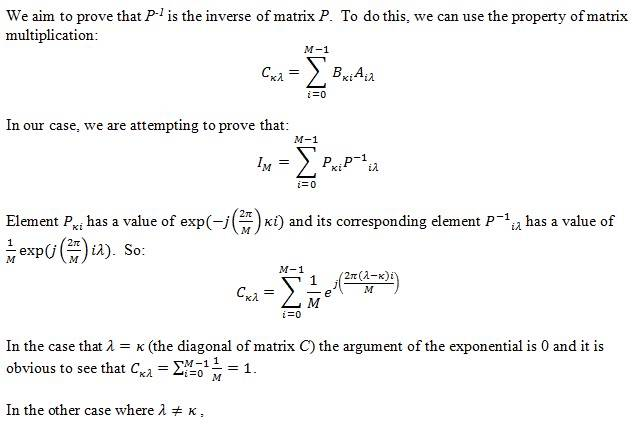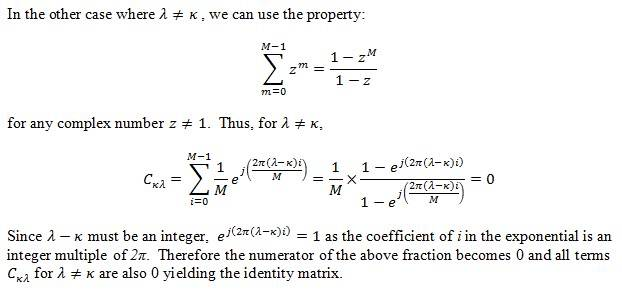# Proving Inverse Matrices?

swuster

## Homework Statement

I'm trying to show that a given matrix is the inverse of the other, by showing that multiplying them together generates the identity matrix. I can't see a way to simplify the last step and I feel like I'm missing something..? Any input on this would be helpful. Thanks!

see below
j = sqrt(-1)

## The Attempt at a SolutionHow do I prove that the rest of the terms are 0?

## Answers and Replies

Homework Helper
Gold Member
It looks to me like the last sentence, which you cut off at the comma, might give you a hint. What does it say?

swuster
It's not cut off. It's as far as I have gotten with the proof, haha. There is nothing obvious that jumps out at me when I consider the case where the exponential argument isn't 0.

Homework Helper
Gold Member
Try using

$$\sum_{m=0}^{M-1} z^m = \frac{1 - z^{M}}{1 - z}$$

which holds for any complex number $z \neq 1$.

swuster
Thanks so much! That did the trick!Homework Helper
Gold Member
Thanks so much! That did the trick!Looks good, nice job.

It's also insightful to consider what is going on geometrically.

This sum:

$$\sum_{i=0}^{M-1}\frac{1}{M}e^{j\left(\frac{2\pi(\lambda-\kappa)i}{M}\right)$$

is calculating the average of $M$ complex numbers. Furthermore, since $\lambda - \kappa$ is a nonzero integer, these complex numbers are evenly spaced samples around the unit circle, so their average is zero.